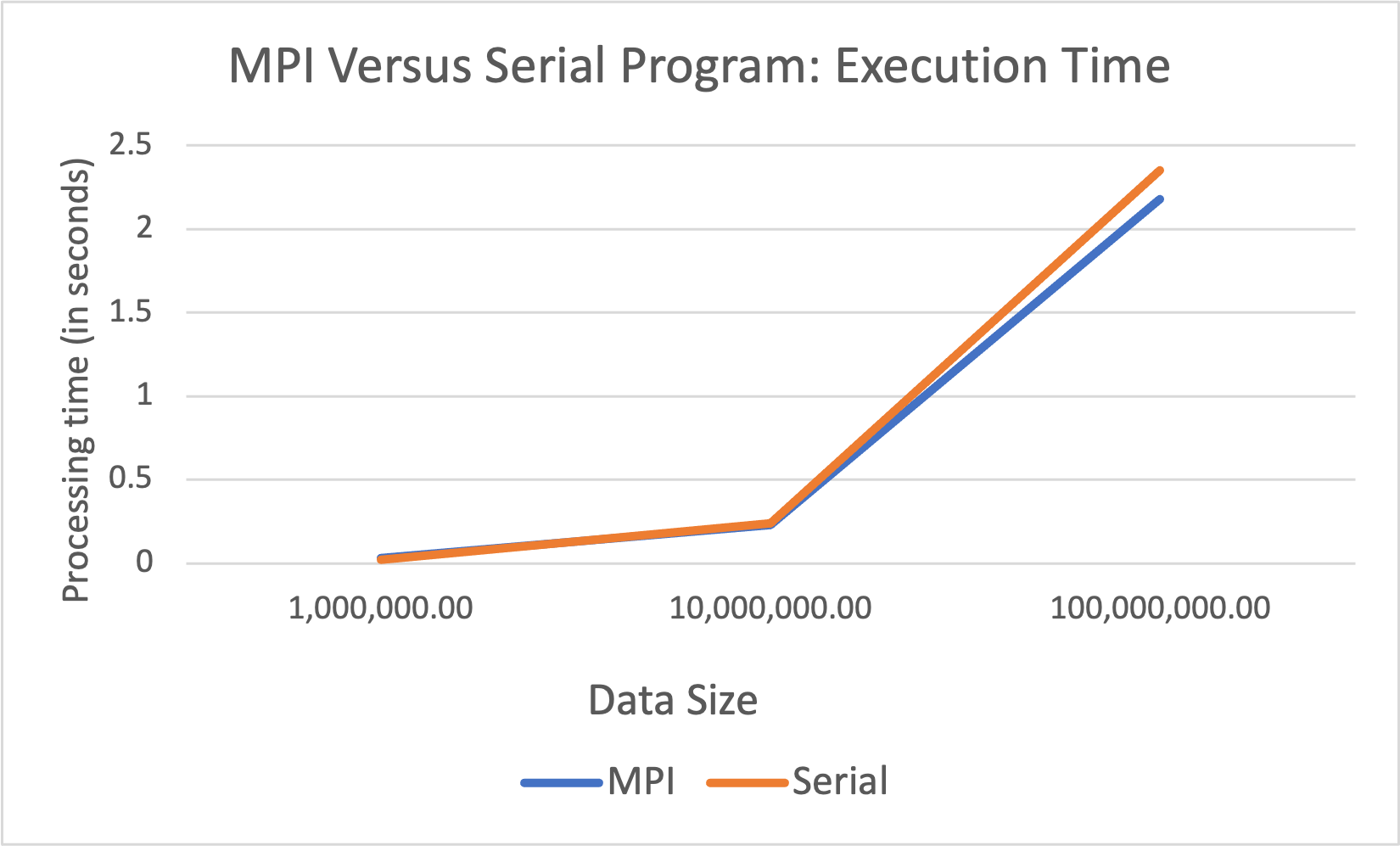# Serial Versus Parallel Programs

This section aims at showing the benefit of using MPI parallel applications over serial applications for some tasks. Here, the performance of an MPI parallel program that sequentially finds a number in a list to compute its frequency is evaluated against the serial version of the program. As for the evaluation metrics, the execution time of running both programs over different data sizes (number of elements in the list) is collected. in detail, the parallel version of the program performs as follows:

• Creates four processes.

• The master process splits the list of numbers into equal parts and distributes them between the worker processes along with the desired number.

• Each process computes the frequency of the number in its part of the list and sends the work result to the master process.

• The master process collects the work results, sums them up, and prints out the total frequency.

Below are the source code sections of the parallel and the serial versions of the program.

• C program sequential_search.c

``````void serial_sequential_search(int num, int list_of_numbers[])
{
int i;
time_t t;

// Use current time as seed for random generator
srand((unsigned) time(&t));

// compute the frequancy of the user input
unsigned long frequancy = 0;
for(i = 0; i < ARRAY_SIZE; ++i)
if(list_of_numbers[i] == num)
frequancy += 1;

// print out the frequancy
printf("The frequancy of %d in the list of numbers is %ld\n", num, frequancy);
}``````
• C program mpi_sequential_search.c

``````void parallel_sequential_search(int num, int list_of_numbers[])
{
int i;
time_t t;

MPI_Status status;

// Initialize the MPI environment
MPI_Init(NULL, NULL);

// Get the rank of the process
int pid;
MPI_Comm_rank(MPI_COMM_WORLD, &pid);

// Get the number of processes
int number_of_processes;
MPI_Comm_size(MPI_COMM_WORLD, &number_of_processes);

if (pid == 0) {
// master process

int index, i;
int elements_per_process;
unsigned long frequency;

elements_per_process = ARRAY_SIZE / number_of_processes;

// check if more than 1 processes are running
if (number_of_processes > 1) {
// distributes the portion of the array among all processes
for (i = 1; i < number_of_processes - 1; i++) {
index = i * elements_per_process;

MPI_Send(&elements_per_process,
1, MPI_INT, i, 0,
MPI_COMM_WORLD);
MPI_Send(&list_of_numbers[index],
elements_per_process,
MPI_INT, i, 0,
MPI_COMM_WORLD);
}

// last process adds remaining elements
index = i * elements_per_process;
int elements_left = ARRAY_SIZE - index;

MPI_Send(&elements_left,
1, MPI_INT,
i, 0,
MPI_COMM_WORLD);
MPI_Send(&list_of_numbers[index],
elements_left,
MPI_INT, i, 0,
MPI_COMM_WORLD);
}

// master process computes the frequency in its portion of the array
frequency = 0;
for(i = 0; i < elements_per_process; ++i)
if(list_of_numbers[i] == num)
frequency += 1;

// collect partial frequency from other processes
unsigned long buffer = 0;
for (i = 1; i < number_of_processes; i++) {
MPI_Recv(&buffer, 1, MPI_INT,
MPI_ANY_SOURCE, 0,
MPI_COMM_WORLD,
&status);
frequency += buffer;
}

// print the frequency of user input in the list of numbers
printf("The frequency of %d in the list of numbers is %ld\n", num, frequency);
}
else {
// worker processes

static int buffer[ARRAY_SIZE];
int num_of_elements_recieved = 0;
unsigned long frequency = 0;

MPI_Recv(&num_of_elements_recieved,
1, MPI_INT, 0, 0,
MPI_COMM_WORLD,
&status);

// store the received portion of the array in buffer
MPI_Recv(&buffer, num_of_elements_recieved,
MPI_INT, 0, 0,
MPI_COMM_WORLD,
&status);

// compute the frequency in received portion of the array
for(i = 0; i < num_of_elements_recieved; ++i)
if(buffer[i] == num)
frequency += 1;

// send the computation result to the master process
MPI_Send(&frequency, 1, MPI_INT,
0, 0, MPI_COMM_WORLD);
}

// Finalize the MPI environment
MPI_Finalize();
}``````

## Experimental ResultsThe line graph above compares the performance of both the serial program and its MPI parallel version. Here, the x-axis represents the size of the array, and the y-axis represents the execution time every program spent to perform a linear search over each data size. From the graph, we can conclude that the serial program performs better by completing its task faster compared to the MPI parallel program when having small data sizes. It’s most likely because of the overheads of initializing, managing, and terminating the MPI execution environment. However, as the data grows in size, the execution time of the serial program starts to increase while the execution time of the MPI parallel program decreases. This shows that the MPI parallel program significantly performs better than the serial program on large data sets.